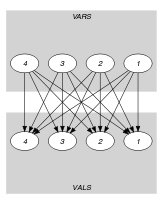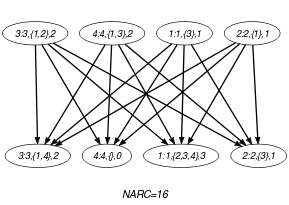## 5.399. symmetric_gcc

Origin

Derived from $\mathrm{𝚐𝚕𝚘𝚋𝚊𝚕}_\mathrm{𝚌𝚊𝚛𝚍𝚒𝚗𝚊𝚕𝚒𝚝𝚢}$ by W. Kocjan.

Constraint

$\mathrm{𝚜𝚢𝚖𝚖𝚎𝚝𝚛𝚒𝚌}_\mathrm{𝚐𝚌𝚌}\left(\mathrm{𝚅𝙰𝚁𝚂},\mathrm{𝚅𝙰𝙻𝚂}\right)$

Synonym

$\mathrm{𝚜𝚐𝚌𝚌}$.

Arguments
 $\mathrm{𝚅𝙰𝚁𝚂}$ $\mathrm{𝚌𝚘𝚕𝚕𝚎𝚌𝚝𝚒𝚘𝚗}\left(\mathrm{𝚒𝚍𝚟𝚊𝚛}-\mathrm{𝚒𝚗𝚝},\mathrm{𝚟𝚊𝚛}-\mathrm{𝚜𝚟𝚊𝚛},\mathrm{𝚗𝚘𝚌𝚌}-\mathrm{𝚍𝚟𝚊𝚛}\right)$ $\mathrm{𝚅𝙰𝙻𝚂}$ $\mathrm{𝚌𝚘𝚕𝚕𝚎𝚌𝚝𝚒𝚘𝚗}\left(\mathrm{𝚒𝚍𝚟𝚊𝚕}-\mathrm{𝚒𝚗𝚝},\mathrm{𝚟𝚊𝚕}-\mathrm{𝚜𝚟𝚊𝚛},\mathrm{𝚗𝚘𝚌𝚌}-\mathrm{𝚍𝚟𝚊𝚛}\right)$
Restrictions
 $\mathrm{𝚛𝚎𝚚𝚞𝚒𝚛𝚎𝚍}$$\left(\mathrm{𝚅𝙰𝚁𝚂},\left[\mathrm{𝚒𝚍𝚟𝚊𝚛},\mathrm{𝚟𝚊𝚛},\mathrm{𝚗𝚘𝚌𝚌}\right]\right)$ $|\mathrm{𝚅𝙰𝚁𝚂}|\ge 1$ $\mathrm{𝚅𝙰𝚁𝚂}.\mathrm{𝚒𝚍𝚟𝚊𝚛}\ge 1$ $\mathrm{𝚅𝙰𝚁𝚂}.\mathrm{𝚒𝚍𝚟𝚊𝚛}\le |\mathrm{𝚅𝙰𝚁𝚂}|$ $\mathrm{𝚍𝚒𝚜𝚝𝚒𝚗𝚌𝚝}$$\left(\mathrm{𝚅𝙰𝚁𝚂},\mathrm{𝚒𝚍𝚟𝚊𝚛}\right)$ $\mathrm{𝚅𝙰𝚁𝚂}.\mathrm{𝚗𝚘𝚌𝚌}\ge 0$ $\mathrm{𝚅𝙰𝚁𝚂}.\mathrm{𝚗𝚘𝚌𝚌}\le |\mathrm{𝚅𝙰𝙻𝚂}|$ $\mathrm{𝚛𝚎𝚚𝚞𝚒𝚛𝚎𝚍}$$\left(\mathrm{𝚅𝙰𝙻𝚂},\left[\mathrm{𝚒𝚍𝚟𝚊𝚕},\mathrm{𝚟𝚊𝚕},\mathrm{𝚗𝚘𝚌𝚌}\right]\right)$ $|\mathrm{𝚅𝙰𝙻𝚂}|\ge 1$ $\mathrm{𝚅𝙰𝙻𝚂}.\mathrm{𝚒𝚍𝚟𝚊𝚕}\ge 1$ $\mathrm{𝚅𝙰𝙻𝚂}.\mathrm{𝚒𝚍𝚟𝚊𝚕}\le |\mathrm{𝚅𝙰𝙻𝚂}|$ $\mathrm{𝚍𝚒𝚜𝚝𝚒𝚗𝚌𝚝}$$\left(\mathrm{𝚅𝙰𝙻𝚂},\mathrm{𝚒𝚍𝚟𝚊𝚕}\right)$ $\mathrm{𝚅𝙰𝙻𝚂}.\mathrm{𝚗𝚘𝚌𝚌}\ge 0$ $\mathrm{𝚅𝙰𝙻𝚂}.\mathrm{𝚗𝚘𝚌𝚌}\le |\mathrm{𝚅𝙰𝚁𝚂}|$
Purpose

Put in relation two sets: for each element of one set gives the corresponding elements of the other set to which it is associated. In addition, enforce a cardinality constraint on the number of occurrences of each value.

Example
$\left(\begin{array}{c}〈\begin{array}{ccc}\mathrm{𝚒𝚍𝚟𝚊𝚛}-1\hfill & \mathrm{𝚟𝚊𝚛}-\left\{3\right\}\hfill & \mathrm{𝚗𝚘𝚌𝚌}-1,\hfill \\ \mathrm{𝚒𝚍𝚟𝚊𝚛}-2\hfill & \mathrm{𝚟𝚊𝚛}-\left\{1\right\}\hfill & \mathrm{𝚗𝚘𝚌𝚌}-1,\hfill \\ \mathrm{𝚒𝚍𝚟𝚊𝚛}-3\hfill & \mathrm{𝚟𝚊𝚛}-\left\{1,2\right\}\hfill & \mathrm{𝚗𝚘𝚌𝚌}-2,\hfill \\ \mathrm{𝚒𝚍𝚟𝚊𝚛}-4\hfill & \mathrm{𝚟𝚊𝚛}-\left\{1,3\right\}\hfill & \mathrm{𝚗𝚘𝚌𝚌}-2\hfill \end{array}〉,\hfill \\ 〈\begin{array}{ccc}\mathrm{𝚒𝚍𝚟𝚊𝚕}-1\hfill & \mathrm{𝚟𝚊𝚕}-\left\{2,3,4\right\}\hfill & \mathrm{𝚗𝚘𝚌𝚌}-3,\hfill \\ \mathrm{𝚒𝚍𝚟𝚊𝚕}-2\hfill & \mathrm{𝚟𝚊𝚕}-\left\{3\right\}\hfill & \mathrm{𝚗𝚘𝚌𝚌}-1,\hfill \\ \mathrm{𝚒𝚍𝚟𝚊𝚕}-3\hfill & \mathrm{𝚟𝚊𝚕}-\left\{1,4\right\}\hfill & \mathrm{𝚗𝚘𝚌𝚌}-2,\hfill \\ \mathrm{𝚒𝚍𝚟𝚊𝚕}-4\hfill & \mathrm{𝚟𝚊𝚕}-\varnothing \hfill & \mathrm{𝚗𝚘𝚌𝚌}-0\hfill \end{array}〉\hfill \end{array}\right)$

The $\mathrm{𝚜𝚢𝚖𝚖𝚎𝚝𝚛𝚒𝚌}_\mathrm{𝚐𝚌𝚌}$ constraint holds since:

• $3\in \mathrm{𝚅𝙰𝚁𝚂}\left[1\right].\mathrm{𝚟𝚊𝚛}⇔1\in \mathrm{𝚅𝙰𝙻𝚂}\left[3\right].\mathrm{𝚟𝚊𝚕}$,

• $1\in \mathrm{𝚅𝙰𝚁𝚂}\left[2\right].\mathrm{𝚟𝚊𝚛}⇔2\in \mathrm{𝚅𝙰𝙻𝚂}\left[1\right].\mathrm{𝚟𝚊𝚕}$,

• $1\in \mathrm{𝚅𝙰𝚁𝚂}\left[3\right].\mathrm{𝚟𝚊𝚛}⇔3\in \mathrm{𝚅𝙰𝙻𝚂}\left[1\right].\mathrm{𝚟𝚊𝚕}$,

• $2\in \mathrm{𝚅𝙰𝚁𝚂}\left[3\right].\mathrm{𝚟𝚊𝚛}⇔3\in \mathrm{𝚅𝙰𝙻𝚂}\left[2\right].\mathrm{𝚟𝚊𝚕}$,

• $1\in \mathrm{𝚅𝙰𝚁𝚂}\left[4\right].\mathrm{𝚟𝚊𝚛}⇔4\in \mathrm{𝚅𝙰𝙻𝚂}\left[1\right].\mathrm{𝚟𝚊𝚕}$,

• $3\in \mathrm{𝚅𝙰𝚁𝚂}\left[4\right].\mathrm{𝚟𝚊𝚛}⇔4\in \mathrm{𝚅𝙰𝙻𝚂}\left[3\right].\mathrm{𝚟𝚊𝚕}$,

• The number of elements of $\mathrm{𝚅𝙰𝚁𝚂}\left[1\right].\mathrm{𝚟𝚊𝚛}=\left\{3\right\}$ is equal to 1,

• The number of elements of $\mathrm{𝚅𝙰𝚁𝚂}\left[2\right].\mathrm{𝚟𝚊𝚛}=\left\{1\right\}$ is equal to 1,

• The number of elements of $\mathrm{𝚅𝙰𝚁𝚂}\left[3\right].\mathrm{𝚟𝚊𝚛}=\left\{1,2\right\}$ is equal to 2,

• The number of elements of $\mathrm{𝚅𝙰𝚁𝚂}\left[4\right].\mathrm{𝚟𝚊𝚛}=\left\{1,3\right\}$ is equal to 2,

• The number of elements of $\mathrm{𝚅𝙰𝙻𝚂}\left[1\right].\mathrm{𝚟𝚊𝚕}=\left\{2,3,4\right\}$ is equal to 3,

• The number of elements of $\mathrm{𝚅𝙰𝙻𝚂}\left[2\right].\mathrm{𝚟𝚊𝚕}=\left\{3\right\}$ is equal to 1,

• The number of elements of $\mathrm{𝚅𝙰𝙻𝚂}\left[3\right].\mathrm{𝚟𝚊𝚕}=\left\{1,4\right\}$ is equal to 2,

• The number of elements of $\mathrm{𝚅𝙰𝙻𝚂}\left[4\right].\mathrm{𝚟𝚊𝚕}=\varnothing$ is equal to 0.

Typical
 $|\mathrm{𝚅𝙰𝚁𝚂}|>1$ $|\mathrm{𝚅𝙰𝙻𝚂}|>1$
Symmetries
• Items of $\mathrm{𝚅𝙰𝚁𝚂}$ are permutable.

• Items of $\mathrm{𝚅𝙰𝙻𝚂}$ are permutable.

Usage

The most simple example of applying $\mathrm{𝚜𝚢𝚖𝚖𝚎𝚝𝚛𝚒𝚌}_\mathrm{𝚐𝚌𝚌}$ is a variant of personnel assignment problem, where one person can be assigned to perform between $n$ and $m$ $\left(n\le m\right)$ jobs, and every job requires between $p$ and $q$ $\left(p\le q\right)$ persons. In addition every job requires different kind of skills. The previous problem can be modelled as follows:

• For each person we create an item of the $\mathrm{𝚅𝙰𝚁𝚂}$ collection,

• For each job we create an item of the $\mathrm{𝚅𝙰𝙻𝚂}$ collection,

• There is an arc between a person and the particular job if this person is qualified to perform it.

Remark

The $\mathrm{𝚜𝚢𝚖𝚖𝚎𝚝𝚛𝚒𝚌}_\mathrm{𝚐𝚌𝚌}$ constraint generalises the $\mathrm{𝚐𝚕𝚘𝚋𝚊𝚕}_\mathrm{𝚌𝚊𝚛𝚍𝚒𝚗𝚊𝚕𝚒𝚝𝚢}$ constraint by allowing a variable to take more than one value. It corresponds to a variant of the $\mathrm{𝚜𝚢𝚖𝚖𝚎𝚝𝚛𝚒𝚌}_\mathrm{𝚌𝚊𝚛𝚍𝚒𝚗𝚊𝚕𝚒𝚝𝚢}$ constraint described in [KocjanKreuger04] where the occurrence variables of the $\mathrm{𝚅𝙰𝚁𝚂}$ and $\mathrm{𝚅𝙰𝙻𝚂}$ collections are replaced by fixed intervals.

specialisation: $\mathrm{𝚜𝚢𝚖𝚖𝚎𝚝𝚛𝚒𝚌}_\mathrm{𝚌𝚊𝚛𝚍𝚒𝚗𝚊𝚕𝚒𝚝𝚢}$ ($\mathrm{𝚟𝚊𝚛𝚒𝚊𝚋𝚕𝚎}$ replaced by $\mathrm{𝚏𝚒𝚡𝚎𝚍}$ $\mathrm{𝚒𝚗𝚝𝚎𝚛𝚟𝚊𝚕}$).

Keywords
Arc input(s)

$\mathrm{𝚅𝙰𝚁𝚂}$ $\mathrm{𝚅𝙰𝙻𝚂}$

Arc generator
$\mathrm{𝑃𝑅𝑂𝐷𝑈𝐶𝑇}$$↦\mathrm{𝚌𝚘𝚕𝚕𝚎𝚌𝚝𝚒𝚘𝚗}\left(\mathrm{𝚟𝚊𝚛𝚜},\mathrm{𝚟𝚊𝚕𝚜}\right)$

Arc arity
Arc constraint(s)
 $•$$\mathrm{𝚒𝚗}_\mathrm{𝚜𝚎𝚝}$$\left(\mathrm{𝚟𝚊𝚛𝚜}.\mathrm{𝚒𝚍𝚟𝚊𝚛},\mathrm{𝚟𝚊𝚕𝚜}.\mathrm{𝚟𝚊𝚕}\right)⇔$$\mathrm{𝚒𝚗}_\mathrm{𝚜𝚎𝚝}$$\left(\mathrm{𝚟𝚊𝚕𝚜}.\mathrm{𝚒𝚍𝚟𝚊𝚕},\mathrm{𝚟𝚊𝚛𝚜}.\mathrm{𝚟𝚊𝚛}\right)$ $•\mathrm{𝚟𝚊𝚛𝚜}.\mathrm{𝚗𝚘𝚌𝚌}=\mathrm{𝚌𝚊𝚛𝚍}_\mathrm{𝚜𝚎𝚝}\left(\mathrm{𝚟𝚊𝚛𝚜}.\mathrm{𝚟𝚊𝚛}\right)$ $•\mathrm{𝚟𝚊𝚕𝚜}.\mathrm{𝚗𝚘𝚌𝚌}=\mathrm{𝚌𝚊𝚛𝚍}_\mathrm{𝚜𝚎𝚝}\left(\mathrm{𝚟𝚊𝚕𝚜}.\mathrm{𝚟𝚊𝚕}\right)$
Graph property(ies)
$\mathrm{𝐍𝐀𝐑𝐂}$$=|\mathrm{𝚅𝙰𝚁𝚂}|*|\mathrm{𝚅𝙰𝙻𝚂}|$

Graph model

The graph model used for the $\mathrm{𝚜𝚢𝚖𝚖𝚎𝚝𝚛𝚒𝚌}_\mathrm{𝚐𝚌𝚌}$ is similar to the one used in the $\mathrm{𝚍𝚘𝚖𝚊𝚒𝚗}_\mathrm{𝚌𝚘𝚗𝚜𝚝𝚛𝚊𝚒𝚗𝚝}$ or in the $\mathrm{𝚕𝚒𝚗𝚔}_\mathrm{𝚜𝚎𝚝}_\mathrm{𝚝𝚘}_\mathrm{𝚋𝚘𝚘𝚕𝚎𝚊𝚗𝚜}$ constraints: we use an equivalence in the arc constraint and ask all arc constraints to hold.

Parts (A) and (B) of Figure 5.399.1 respectively show the initial and final graph. Since we use the $\mathrm{𝐍𝐀𝐑𝐂}$ graph property, all the arcs of the final graph are stressed in bold.

##### Figure 5.399.1. Initial and final graph of the $\mathrm{𝚜𝚢𝚖𝚖𝚎𝚝𝚛𝚒𝚌}_\mathrm{𝚐𝚌𝚌}$ constraint(a) (b)
Signature

Since we use the $\mathrm{𝑃𝑅𝑂𝐷𝑈𝐶𝑇}$ arc generator on the collections $\mathrm{𝚅𝙰𝚁𝚂}$ and $\mathrm{𝚅𝙰𝙻𝚂}$, the number of arcs of the initial graph is equal to $|\mathrm{𝚅𝙰𝚁𝚂}|·|\mathrm{𝚅𝙰𝙻𝚂}|$. Therefore the maximum number of arcs of the final graph is also equal to $|\mathrm{𝚅𝙰𝚁𝚂}|·|\mathrm{𝚅𝙰𝙻𝚂}|$ and we can rewrite $\mathrm{𝐍𝐀𝐑𝐂}=|\mathrm{𝚅𝙰𝚁𝚂}|·|\mathrm{𝚅𝙰𝙻𝚂}|$ to $\mathrm{𝐍𝐀𝐑𝐂}\ge |\mathrm{𝚅𝙰𝚁𝚂}|·|\mathrm{𝚅𝙰𝙻𝚂}|$. So we can simplify $\underline{\overline{\mathrm{𝐍𝐀𝐑𝐂}}}$ to $\overline{\mathrm{𝐍𝐀𝐑𝐂}}$.